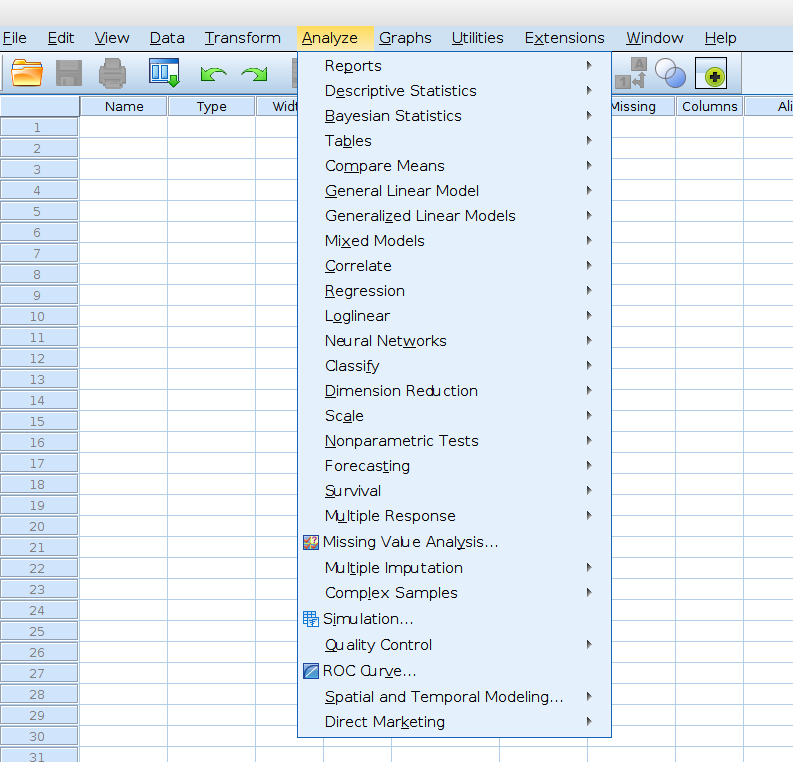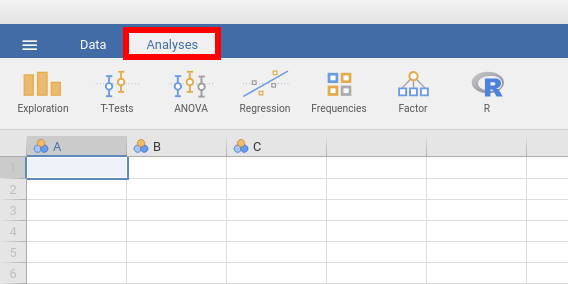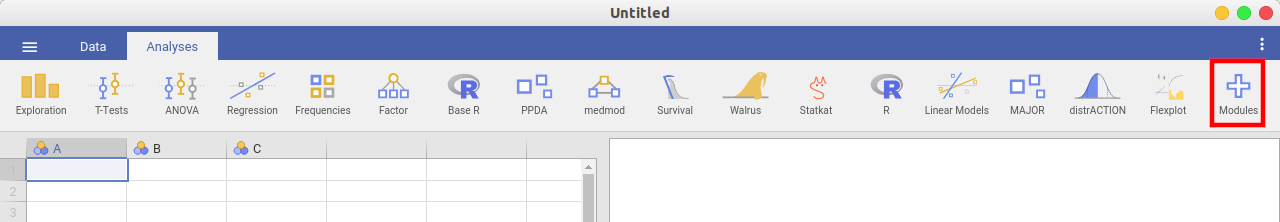# Comparison of analyses available in SPSS and jamovi

 SPSS (version 27) The overview covers functions from SPSS Base (99\$) and Advanced (79 US\$), i.e. 178 US\$ / user and month. jamovi (versjon 2.0)Already at first glance, it becomes clear that jamovi currently has fewer features than SPSS. But: (1) There is a (ever increasing) made available via modules (press the “+” sign in the right upper corner of the jamovi window to add them) and (2) The features implemented already cover “standard” needs (90% of the most common analyses used in psychology). Feel free to check out which modules are available: There is also quite a wealth of modules covering functions that are not available in SPSS but very useful (e.g., for meta-analyses; MAJOR). If you are willing to use some R code (e.g., in conjunction with the jamovi-module Rj) then you can (most presumably) do every analysis you could imagine.Reports Reports → Codebook N/A Reports → OLAP Cubes N/A Reports → Case summaries Exploration → Descriptives has the same functionality Reports → Reports Summaries in Rows N/A Reports → Reports Summaries in Columns N/A Descriptive Statistics Descriptive Statistics → Frequencies Exploration → Descriptives combines all three procedures tick «Frequency tables» to get an output that is similar to that of «Frequencies» in SPSS Descriptive Statistics → Descriptives Descriptive Statistics → Explore Descriptive Statistics → Crosstabs Frequencies → (Contingency tables) → Independent samples Descriptive Statistics → Ratio N/A Bayesian Statistics requires the jamovi-module «jsq» Bayesian Statistics → One Sample Normal T-Test → Bayesian One Sample T-Test Bayesian Statistics → One Sample Binomial Frequencies → Bayesian Proportion Test Bayesian Statistics → One Sample Poisson Frequencies → Bayesian Contingency Tables Bayesian Statistics → Related Sample Normal T-Test → Bayesian Paired Samples T-Test Bayesian Statistics → Independent Samples Normal T-Test → Bayesian Independent Samples T-Test Bayesian Statistics → Pearson Correlation Regression → Bayesian Correlation Matrix / Bayesian Correlation Pairs Bayesian Statistics → Linear Regression Regression → Bayesian Linear Regression Bayesian Statistics → One-way ANOVA ANOVA → Bayesian ANOVA (can handle several factors while SPSS is limited to one factor) Bayesian Statistics → Log-Linear Models Frequencies → Bayesian Log-Linear Regression Compare Means Compare Means → Means… Exploration → Descriptives replaces / integrates that functionality, choose the drop-down menu «Statistics» and set ticks at «Mean», «N» and «Std. deviation» Compare Means → Independent-Samples T Test T-Test → Independent Samples T-Test Compare Means → Paired-Samples T Test T-Test → Paired Samples T-Test Compare Means → One-Sample T Test T-Test → One Sample T-Test Compare Means → One-Way ANOVA ANOVA → One-Way ANOVA General Linear Model General Linear Model → Univariate ANOVA → One-Way ANOVA General Linear Model → Multivariate ANOVA → MANCOVA General Linear Model → Repeated Measures ANOVA → Repeated Measures ANOVA General Linear Model → Variance Components N/A Generalized Linear Models requires the jamovi-module «GAMLj» (General Analyses for the Linear Model in jamovi) Generalized Linear Models → Generalized Linear Models Generalized Linear Models → Generalized Estimating Equations Mixed Models requires the jamovi-module «GAMLj» (General Analyses for the Linear Model in jamovi) Mixed Models → Linear Mixed Models → Generalized Linear Correlate Correlate → Bivariate Regression → Correlation Matrix Correlate → Partial Regression → Partial Correlation Correlate → Distances N/A Regression Regression → Automatic Linear Models N/A Regression → Linear Regression → Linear Regression Regression → Ordinal Regression → (Logistic Regression) → Ordinal Outcomes Regression → Curve Estimation Regression → Partial Least Squares Loglinear Loglinear → General Frequencies → Log-Linear Regression Loglinear → Logit Loglinear → Model Selection Classify Classify → Nearest Neighbor N/A Classify → Discriminant N/A, can be calculated using R-code and the R-library «MASS» Classify → TwoStep Cluster N/A Classify → Hierarchical Cluster N/A, can be calculated using R-code and the R-library «pvclust» Classify → K-Means Cluster Dimension Reduction Dimension Reduction → Factor Factor → (Data reduction) → Principal Component Analysis Factor → (Data reduction) → Exploratory Factor Analysis 1 Scale Scale → Reliability Analysis Factor → (Scale analysis) → Reliability analysis Scale → Multidimensional Scaling N/A Nonparametric Tests Nonparametric Tests → One Sample N/A, the tests itself are available (see below), but not a common start menu that allows a selection based on your data (e.g., between- or within-subject) Nonparametric Tests → Independent Samples Nonparametric Tests → Related Samples Nonparametric Tests → Legacy Dialogs → Chi-Square Frequencies → (One Sample Proportion Tests) → N Outcomes (x² goodness of fit) Nonparametric Tests → Legacy Dialogs → Binomial Frequencies → (One Sample Proportion Tests) → 2 Outcomes (Binomial test) Nonparametric Tests → Legacy Dialogs → Runs N/A Nonparametric Tests → Legacy Dialogs → 1-Sample K-S N/A, Shapiro-Wilks available under Exploration → Descriptives, choose drop-down menu «Statistics» and tick «Shapiro-Wilks» Nonparametric Tests → Legacy Dialogs → 2 Independent Samples T-Test → Independent Samples T-Test, set tick-box «Mann-Whitney U» Nonparametric Tests → Legacy Dialogs → 2 Related Samples T-Test → Paired Samples T-Test, set tick-box «Wilcoxon Rank» Nonparametric Tests → Legacy Dialogs → K Independent Samples ANOVA → (Non-Parametric) → One-Way ANOVA (Kruskal-Wallis) Nonparametric Tests → Legacy Dialogs → K Related Samples ANOVA → (Non-Parametric) → Repeated Measures ANOVA (Friedman) Survival requires the jamovi-module «Death watch» Survival → Life Tables Survival → Kaplan-Meier Survival → Cox Regression Survival → Cox w/ Time-Dep Cov Multiple Response Multiple Response → Define Variable Sets N/A Multiple Response → Frequencies Multiple Response → Crosstabs ROC Curve ROC Curve N/A, accessible via R packages (e.g., ROCR eller pROC) Simulation Simulation N/A Spatial and Temporal Modeling Spatial and Temporal Modeling → Spatial Modeling N/A
1

Whereas SPSS puts both methods into one procedure (`FACTOR`) makes jamovi a conceptual difference between Principal Component Analysis aiming at data reduction (i.e., reducing the number of dimension that are required to describe the data) and Exploratory Factor Analysis aiming at extracting underlying latent variables.